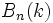# Triangulability theorem

Let$k$ be an algebraically closed field. Denote by$GL_n(k)$ the general linear group of invertible$n \times n$ matrices over$k$ and by$B_n(k)$ the Borel subgroup: the subgroup of invertible upper triangular matrices. Then,$B(n,k)$ is conjugate-dense in$GL(n,k)$. In other words, given any matrix in$GL(n,k)$, we can conjugate it (or change basis) to make it upper triangular.
Note that the result fails over non-algebraically closed fields; in particular, it fails when$k$ is a finite field.